# Engineering Mechanics - Force System Resultants

### Exercise :: Force System Resultants - General Questions

• Force System Resultants - General Questions
11.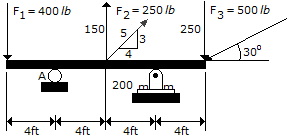Determine the moment of force F3 about point A on the beam.

 A. M3 = 6930 ft-lb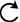B. M3 = 6000 ft-lbC. M3 = 3000 ft-lbD. M3 = 4000 ft-lbExplanation:

No answer description available for this question. Let us discuss.

12.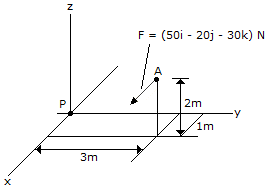Replace the force at A by an equivalent force and couple moment at P. Express the results on Cartesian vector form.

 A. F = (-50i+20j+30k) N, M = (50i-130j+170k) N-m B. F = (-50i+20j+30k) N, M = (-50i+60j+60k) N-m C. F = (50i-20j-30k) N, M = (-50i+130j-170k) N-m D. F = (50i-20j-30k) N, M = (50i-60j-60k) N-m

Explanation:

No answer description available for this question. Let us discuss.

13.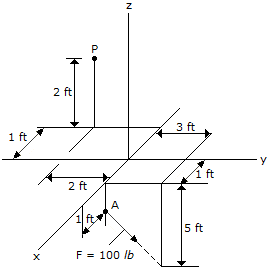Replace the force at A by an equivalent force and couple moment at P. Express the results on Cartesian vector form.

 A. F = (60j-80k) lb, M = (20i+160j+120k) lb-ft B. F = (60j-80k) lb, M = (300j+560k) lb-ft C. F = (60j-80k) lb, M = 20i lb-ft D. F = (60j-80k) lb, M = (-160i-400j+120k) lb-ft

Explanation:

No answer description available for this question. Let us discuss.

14.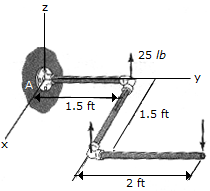A force and couple act on the pipe assembly. Replace this system by an equivalent single resultant force. Specify the location of the resultant force along the y axis, measured from A. The pipe lies in the x-y plane.

 A. R = 25k lb, y = -0.9 ft B. R = 25k lb, y = 0.9 ft C. R = 25k lb, y = 3.9 ft D. R = 25k lb, y = -3.9 ft

Explanation:

No answer description available for this question. Let us discuss.

15.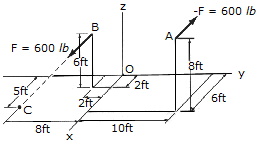Determine the couple moment. Use a vector analysis and express the result as a Cartesian vector.

 A. M = (800i-4800j-800k) lb-ft B. M = (4000i-2000j+4000k) lb-ft C. M = (-4000i+7000j-4000k) lb-ft D. M = (-800i-4800j-800k) lb-ft